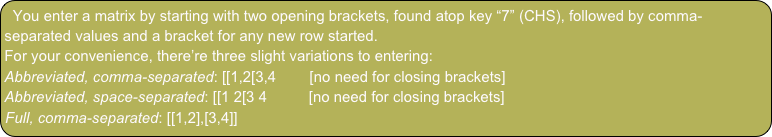Working with MatricesOverviewBasic operators (“+”, “-”, “*”, “/”, ) work with matrices, or matrices in combination with vectors. In addition, there’s a number of specialized functions, found in the Array menu, for tasks like transposing, calculation of norms, trace, rank, determinant.

In this tutorial, we perform some basic operations and use matrices and vectors to solve a linear system of equations.

Basic operations

Let’s begin with some basics.

Enter [[3,4[5,6You’ve entered a 2x2 matrix.

Tap ± (CHS)You inverted it.

Tap Enter to duplicate it.Tap “*”You did a matrix multiplication.

Now, switch to the Array menu.

Tap trans (TRN)     (Classic: first go to the next menu page via tap on → (NEXT))You transposed the matrix.

(Modern: tap → (NEXT) to go to the next menu page)

Tap 2, tap identity (IDN)You created a 2x2 identity matrix.

Tap “+”(Classic: tap → (NEXT) to go to the next menu page)

Tap det (DET)You computed the matrix’s determinant.

Undo. (Tap ⌫ (←))Tap → (NEXT) to go to the next menu page.

Tap rank.You computed the number of linearly independent vectors in your matrix.

There’s more things you can do with matrices. See the Statistics tutorial for more practice with matrix operations, and see the Functions Reference for all the details.

Solving a linear system of equations